# Laws of Exponents: Product and Quotient Rules (Properties of Exponents)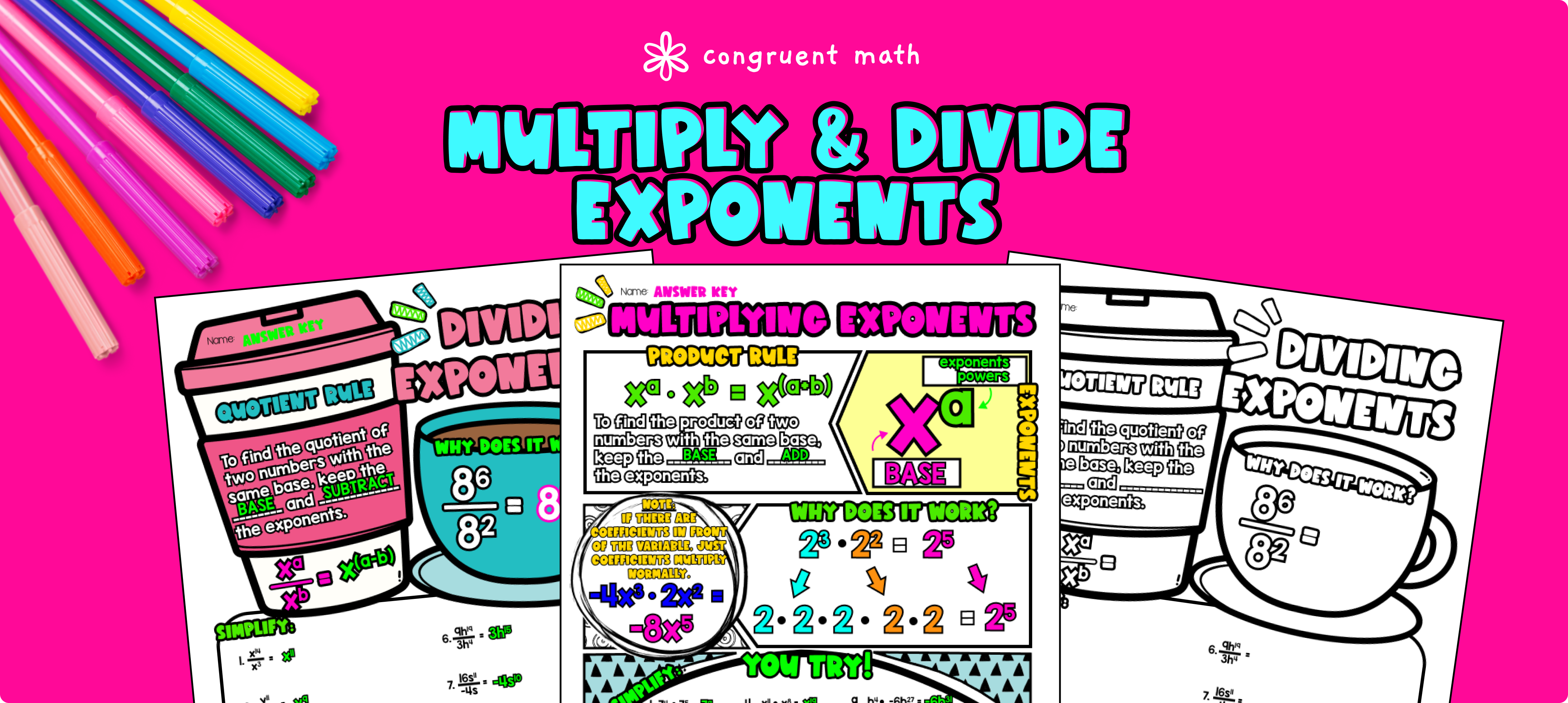Pin This

## Overview

Ever wondered how to teach Laws of Exponents: Multiply & Divide in an engaging way to your 8th grade students?

In this lesson plan, students will learn about the product and quotient rules in the properties of exponents and their real-life applications. Through artistic, interactive guided notes, a maze worksheet, and a doodle & color by number activity, students will gain a comprehensive understanding of the topic.

The lesson culminates with a real-life example that explores how baking desserts requires adjusting recipes using the rules of exponents.

## Get the Lesson Materials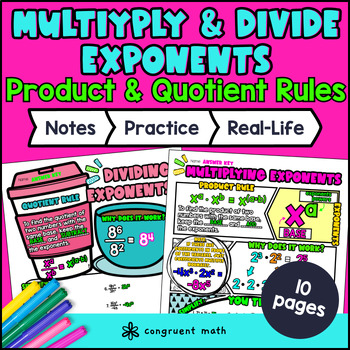\$4.25

## Learning Objectives

After this lesson, students will be able to:

• Define and apply the laws of exponents, specifically product rule and quotient rule to simplify expressions

• Use properties of exponents to multiply and divide exponential terms with the same base

• Explain the real-life applications of the product rule and quotient rules

## Prerequisites

Before this lesson, students should be familiar with:

• Basic algebraic concepts, including variables and expressions

• Basic exponent rules, including the base and power

• How to add and subtract integers

## Key Vocabulary

• Exponent

• Base

• Power

• Product rule

• Quotient rule

• Properties of Exponents

• Laws of Exponents

## Procedure

### Introduction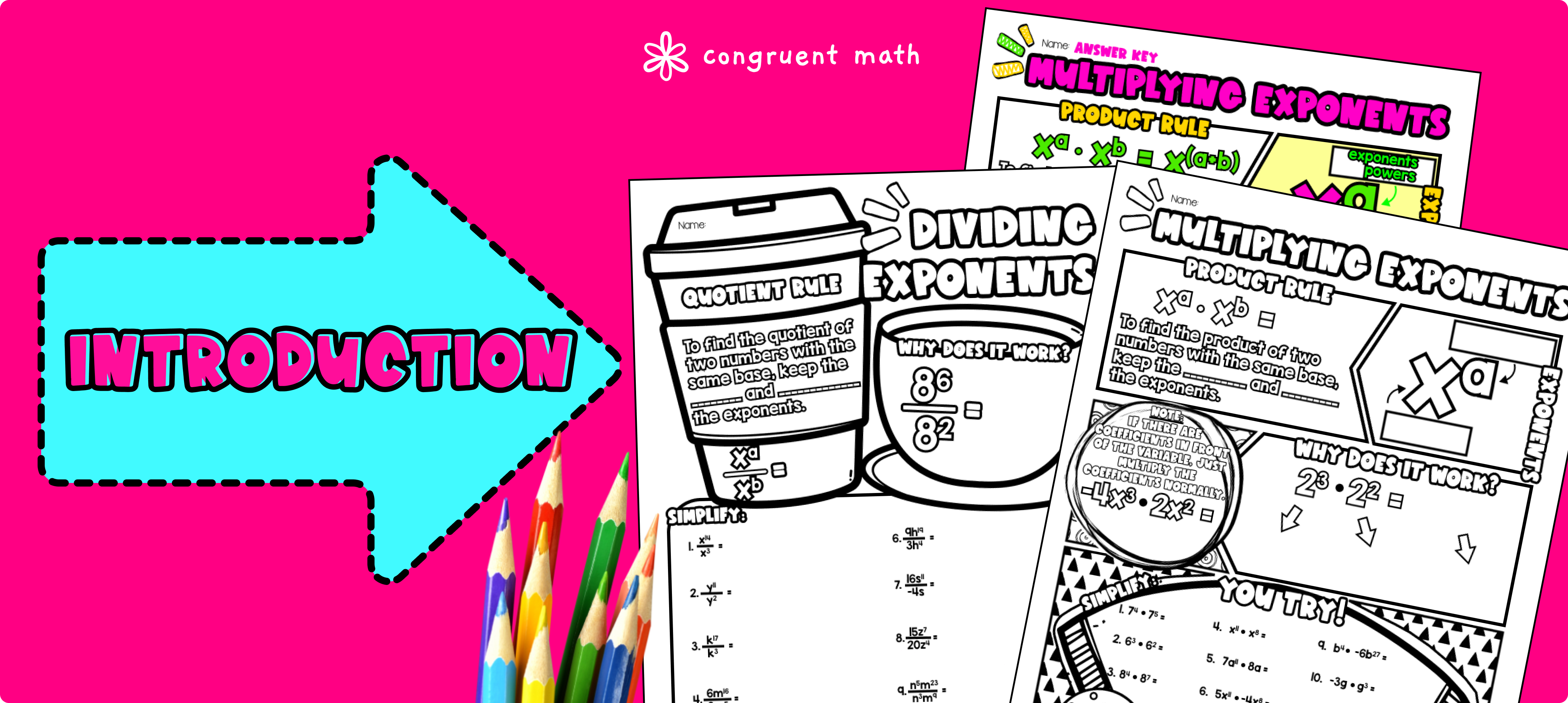Pin This

As a hook, ask students why adjusting recipes with exponents is important when baking desserts.

Use the guided notes to introduce the product and quotient rules in the properties of exponents. Walk through the key points of the topic of the guided notes including keeping the base the same and adding the powers when multiplying exponents, and keeping the base the same and subtracting the powers when dividing exponents.

Refer to the FAQ below for a walk through on this, as well as ideas on how to respond to common student questions. Have students walk through the “You Try!” section at the bottom of the pages. Call on students to talk through their answers, potentially on the whiteboard or projector. Based on student responses, reteach concepts that students need extra help with.

### Practice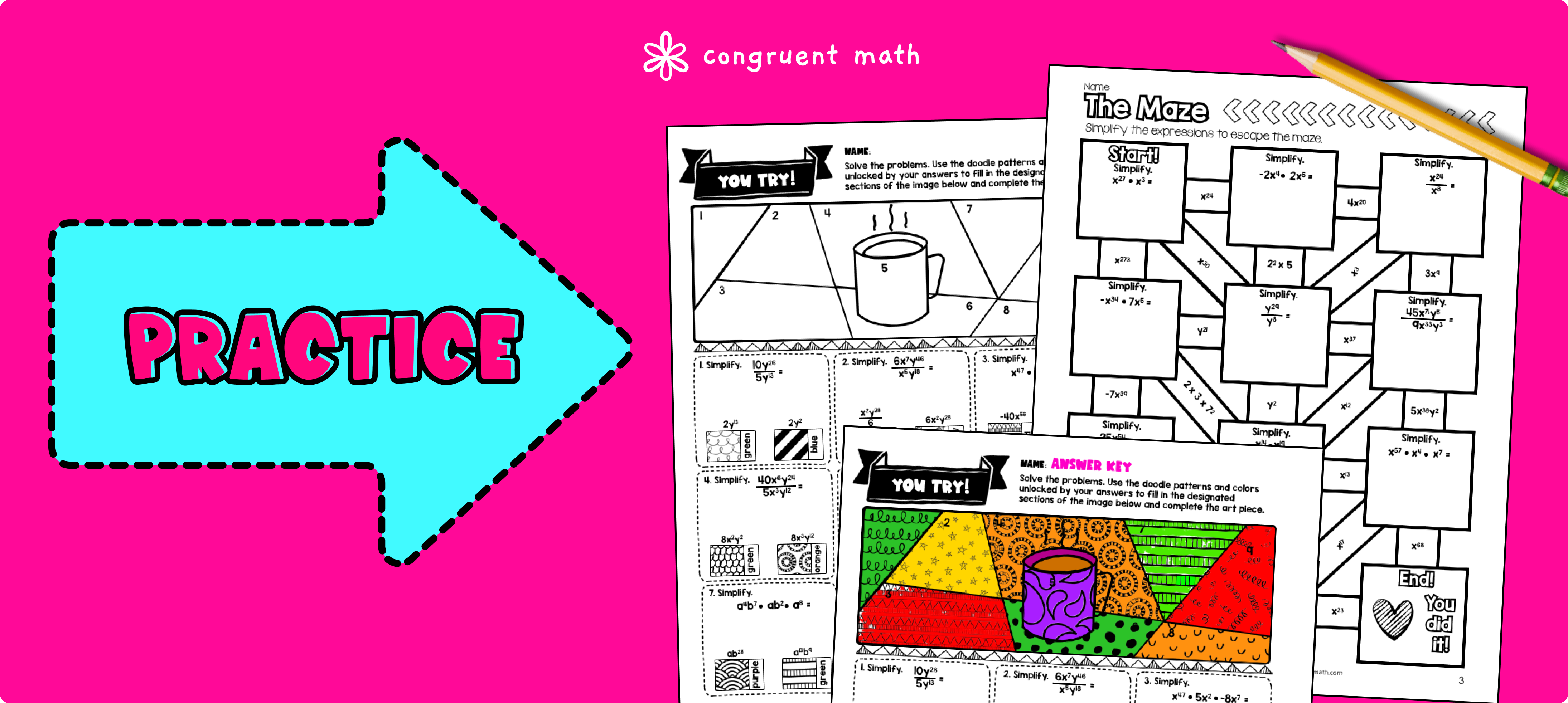Pin This

Have students practice the product and quotient rules using the maze worksheet. Walk around to answer student questions.

Fast finishers can dive into the doodle & color by number activity for extra practice. You can assign it as homework for the remainder of the class.

### Real-Life Application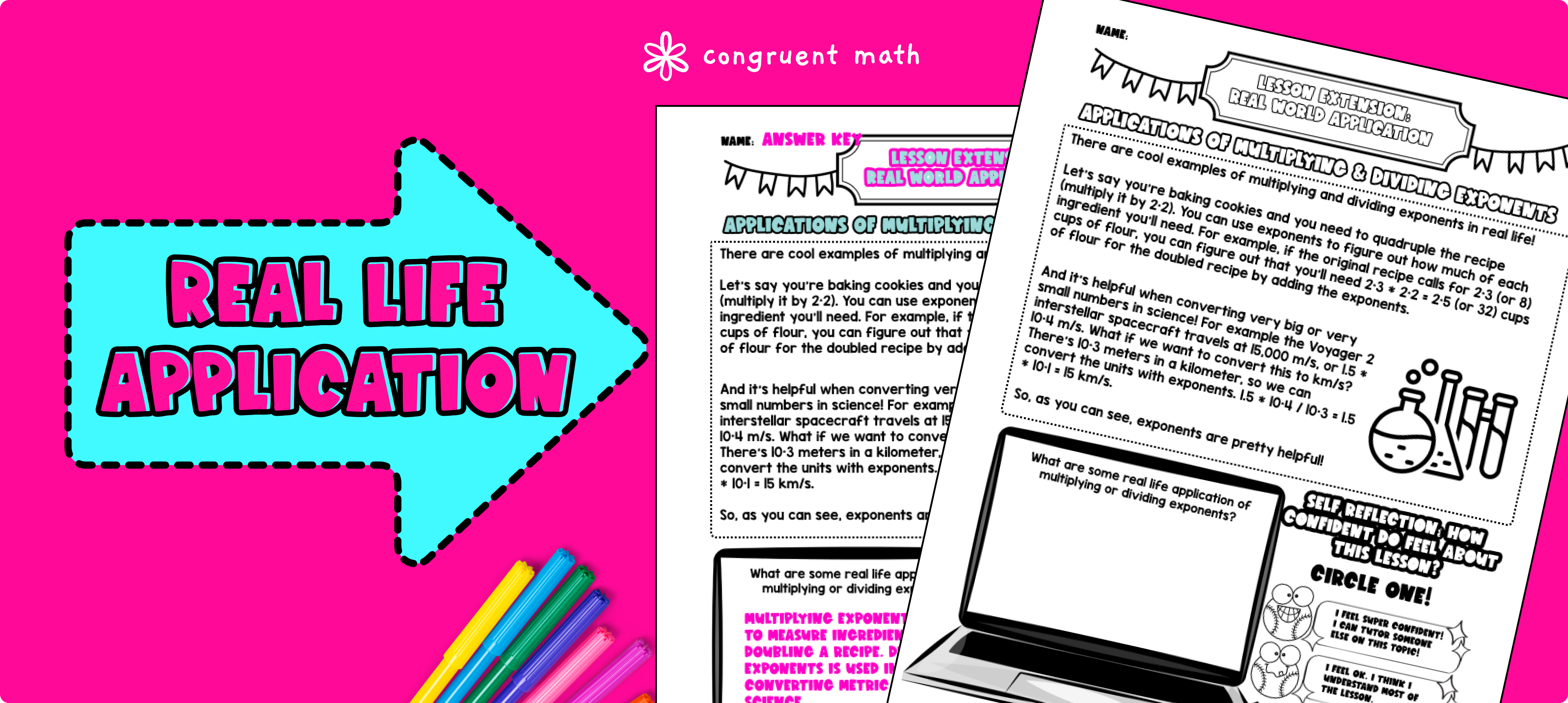Pin This

Bring the class back together, and introduce the concept of adjusting recipes for baking desserts using the rules of exponents. Explain how understanding the product and quotient rules can help in modifying recipes for different serving sizes or portions. You could also bring in examples of recipes that use exponents in their measurements, such as doubling or halving ingredients.

Refer to the FAQ for more ideas on how to teach this real-life application topic.

## Extensions

### Self-Checking Digital Practice Extensions

If you're looking for digital practice for Laws of Exponents: Multiply & Divide, try my Pixel Art activities in Google Sheets. Every answer is automatically checked, and correct answers unlock parts of a mystery picture. It's incredibly fun, and a powerful tool for differentiation.

Here's 1 activity to explore:

### Printable Practice Extensions

A fun, no-prep way to practice the Laws of Exponents is Doodle & Color by Number — they're a fresh take on color by number or color by code. It includes multiple levels of practice, perfect for a review day or sub plan.

Here’s 1 activity to explore.

## Want more ideas and freebies?

Get my free resource library with digital & print activities—plus tips over email.